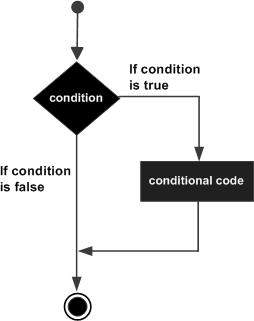# C++ if statement

#### C in Depth: The Complete C Programming Guide for Beginners

45 Lectures 4.5 hours

#### Practical C++: Learn C++ Basics Step by Step

Most Popular

50 Lectures 4.5 hours

#### Master C and Embedded C Programming- Learn as you go

66 Lectures 5.5 hours

An if statement consists of a boolean expression followed by one or more statements.

## Syntax

The syntax of an if statement in C++ is −

```if(boolean_expression) {
// statement(s) will execute if the boolean expression is true
}
```

If the boolean expression evaluates to true, then the block of code inside the if statement will be executed. If boolean expression evaluates to false, then the first set of code after the end of the if statement (after the closing curly brace) will be executed.

## Flow Diagram## Example

```#include <iostream>
using namespace std;

int main () {
// local variable declaration:
int a = 10;

// check the boolean condition
if( a < 20 ) {
// if condition is true then print the following
cout << "a is less than 20;" << endl;
}
cout << "value of a is : " << a << endl;

return 0;
}
```

When the above code is compiled and executed, it produces the following result −

```a is less than 20;
value of a is : 10
```
cpp_decision_making.htm